Select Page

# Important Definitions related to Quantum Computing

by | Jun 27, 2022 | Computing, Quantum Computing

Home » Computing » Quantum Computing » Important Definitions related to Quantum Computing

Preface – This post is part of the Quantum Computing series.

## Introduction

To recap, in the last module, we explored the inner workings of a Quantum Computer by mathematically defining the notion of a Quantum State. We introduced the mathematical notation used to define quantum states and, in the end, discussed the design and operating principles of a D-Wave Quantum Computer.

In this module, we will provide rigorous definitions and corresponding intuition of some technical terms we have encountered in the previous modules.

## Qubit

Qubit is short for Quantum bit. A qubit is a fundamental unit of Quantum Computation. The book Quantum Computation and Quantum Information by Neilson and Chung -which is as close to a standardized textbook on Quantum Computing as we’ve got so far- defines a qubit as an abstract, mathematical object. Although – as some of you may recall from previous modules – a qubit is a physical entity, defining it as an abstract, mathematical object will yield to us the benefit of describing it as a generalized object, independent of its specific, physical method of implementation.

A qubit is the fundamental unit of quantum information. A qubit, just like a classical bit has a state. The state of a qubit is described using Dirac’s notation (“”) which is the standardized notation used to describe quantum states. A qubit can be in states |0, |1⟩, or a linear combination of states |0 and |1and as shown below:

=α|0+β|1

Here,α and β are complex numbers describing probability amplitudes of the qubit being in the |0 or |1 respectively. |0 and |1 are the orthonormal basis vectors in a two-dimensional vector space. Thus, a qubit can be described as a unit vector in a two-dimensional vector space.

Using vector notation, the above equation can be written as: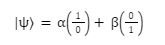## Superposition

Superposition is one of the fundamental principles of Quantum Mechanics. The wave-particle duality describes that an electron behaves as both a wave and a particle, but what does it actually mean? How can something behave as both a wave and a particle? Classically, we know that an object can either be discrete or continuous. But how can it be both? Let’s find out!

#### The Double Slit Experiment

Thomas Young in 1802 designed an experiment to demonstrate the wave nature of light. The Double Slit experiment was designed such that a light source randomly launches photons at a barrier containing two slits as shown in figure.

————–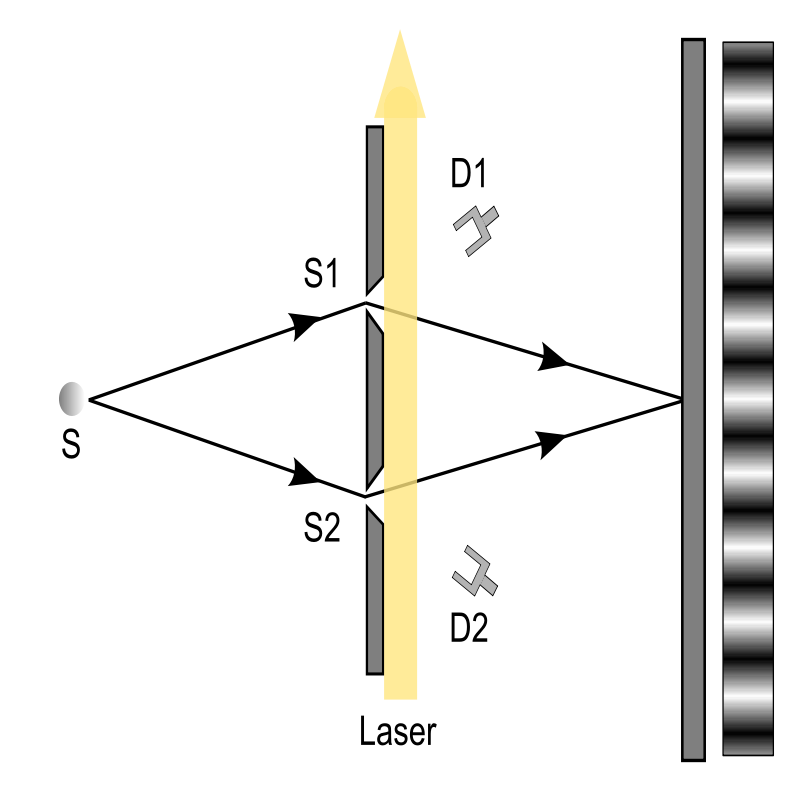Image Source: 老陳, CC BY-SA 4.0, via Wikimedia Commons

The screen behind the barrier should record the pattern which if the photons are assumed to be particles should show something like two vertical lines of light passing through the barrier.

Instead, the screen records an interference pattern as shown below.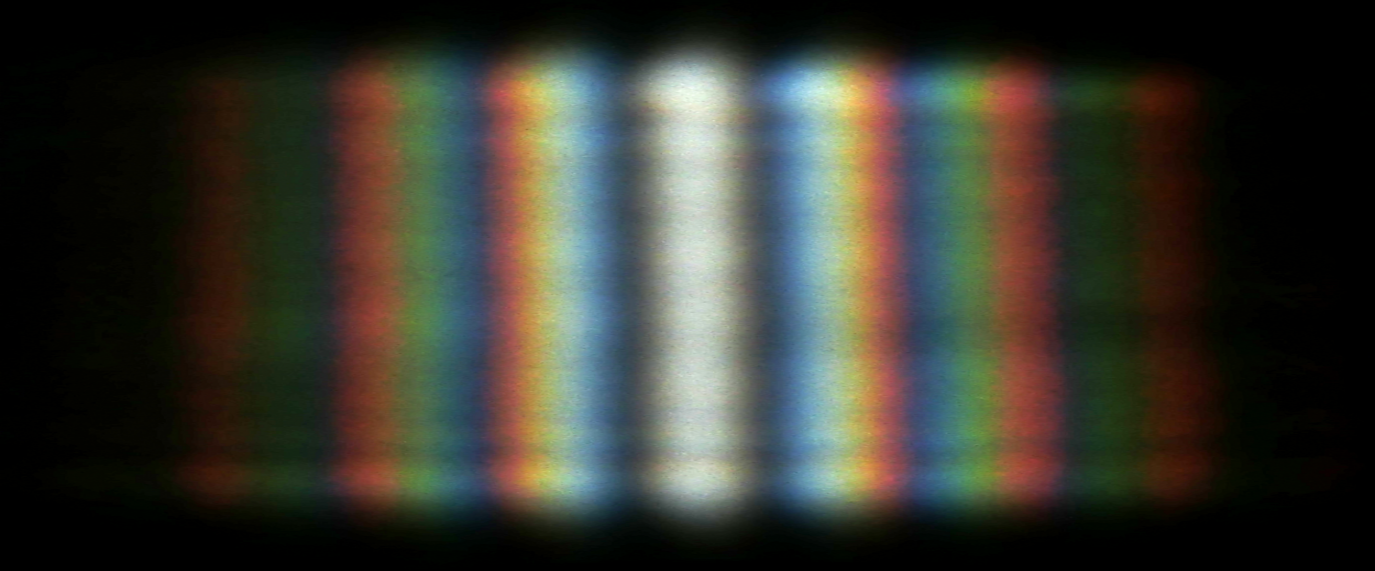Interference patterns are generally generated when waves constructively and destructively interfere with each other. So, although Mr. Young succeeded in demonstrating the wave nature of light no one really understood what was really going on until the theory of Quantum Mechanics was formalized.

So, what on Earth is really going on? Well, the thing is no one really understands what is going on. The path of the photons, the slit they go through and the path they take is not exactly traceable. As we know, measuring a quantum system collapses the superposition, so we cannot detect exactly how photons bring about an interference pattern. But we do know that there must be something fundamentally wave-like in the nature of photons to bring about the interference pattern.

#### Superposition

Later, it was discovered that after going into the slit, the photon exists in superposition. Think of it like a dense cloud, the photon can exist anywhere within the cloud with each position corresponding to a probability amplitude. This is Superposition.

Superposition is defined as the ability of a quantum system to be in a combination of multiple states at the same instance.

#### Measurement

Measurement collapses the superposition. When a quantum system is measured, the superposition collapses and the system comes down to one of its basis states.

#### Two-level superposition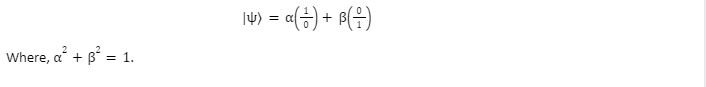#### Four level superposition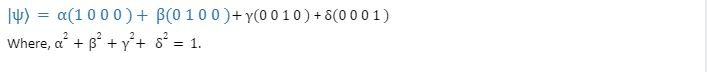## Entanglement

Entanglement is the phenomenon associated with quantum systems where two systems are correlated to each other such that the measurement or manipulation of one system will affect the other immediately even when separated by vast distances.

To understand Entanglement better, let’s take an example. Let’s assume we have two balls that are entangled to each other. The balls are in a superposition of black and white before observation, once observed a ball can either be black or white. Let’s say we have two observers Alice and Bob each observing one ball. The balls are entangled so when Alice observes her ball and sees a black ball Bob will immediately see his ball turn white or vice a versa. (Alice and bob can both see the ball turn the same color as well, it depends upon how the objects are entangled).

Albert Einstein never could reconcile with the theory of entanglement. He referred to entanglement as “spooky”. And it was a perfectly valid reaction as scientists did not know how exactly entanglement worked at the time. How can an object pass information to another object separated by a distance as large as two different galaxies almost immediately? This is only possible if the objects are communicating faster than the speed of light which completely breaks Einstein’s theory of special relativity. This is a common misconception that was later proved false after conducting various experiments. Even Quantum Physics cannot achieve faster than light communication.

## Teleportation

Quantum Teleportation is the process of sending quantum information from a sender to a receiver. Teleportation is achieved through entanglement, the sender and receiver’s qubits are entangled in Bell States. The Bell State is described as follows in a two-qubit system: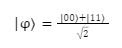Here, in |00 and |11the first 0/1 represents the first qubit and the second 0/1 represents the second qubit. Both the states are in equal superposition with probability amplitude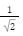: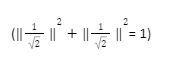Teleportation sends classical information across the channel so it cannot achieve faster than light speed as we discussed in the previous section.

## Author

•A Full Stack Developer with 10+ years of experience in technical content creation.

•